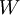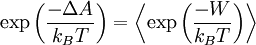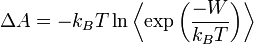# Jarzynski equality

The Jarzynski equality, also known as the work relation or non-equilibrium work relation was developed by Chris Jarzynski. According to this equality, the equilibrium Helmholtz energy function of a process, ($A$), can be reconstructed by averaging the external work,$W$, performed in many non-equilibrium realizations of the process (Eq. 2a in ):$\exp \left( \frac{-\Delta A}{k_BT}\right)= \left\langle \exp \left( \frac{-W}{k_BT} \right) \right\rangle$$\Delta A = - k_BT \ln \left\langle \exp \left( \frac{-W}{k_BT} \right) \right\rangle$
where$k_B$ is the Boltzmann constant and$T$ is the temperature. The only assumption in the proof of this relation is that of a weak coupling between the system and the reservoir. More recently Jarzynski has re-derived this formula, dispensing with this assumption .